# d242: TensorFlow CIFAR-10 tutorial, detailed step-by-step review, Part 1

Detailed step by step review of “Convolutional Neural Networks” TensorFlow CIFAR-10 tutorial (r0.11), Part 1

### Intro

What is cifar-10?
CIFAR-10  is an established computer-vision dataset used for object recognition. It is a subset of the 80 million tiny images dataset and consists of 60,000 32×32 color images containing one of 10 object classes, with 6000 images per class. It was collected by Alex Krizhevsky, Vinod Nair, and Geoffrey Hinton.” https://www.kaggle.com/c/cifar-10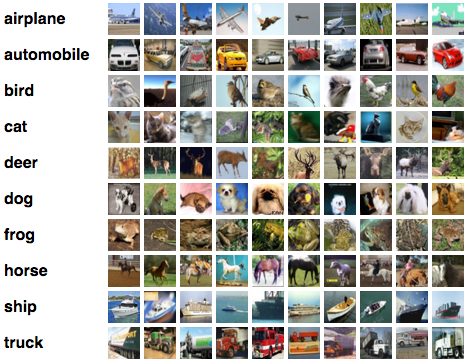“CIFAR-10 classification is a common benchmark problem in machine learning. The problem is to classify RGB 32×32 pixel images across 10 categories: airplane, automobile, bird, cat, deer, dog, frog, horse, ship, and truck.” https://www.tensorflow.org/versions/r0.10/tutorials/deep_cnn/index.html

“The classes are completely mutually exclusive. There is no overlap between automobiles and trucks. “Automobile” includes sedans, SUVs, things of that sort. “Truck” includes only big trucks. Neither includes pickup trucks.” http://www.cs.toronto.edu/~kriz/cifar.html

Original: http://www.cs.toronto.edu/~kriz/cifar.html
Hosted by Kaggle: https://www.kaggle.com/c/cifar-10/data

### Goal of TensorFlow CIFAR-10 tutorial:

Learn TensorFlow and “Building a small convolutional neural network (CNN) for image recognition”

What is Convolutional neural network (CNN)?: Convolutional neural network (convnets) are neural networks that share their parameters across space. Detailed explanation of Convolutional networks:

How convolutional neural network works?

Kashif Rasul – Intro to convolutional neural network:

Learn about image recognitionhttps://www.quora.com/Computer-Vision-What-are-the-best-resources-for-learning-about-image-recognition | Deep learning and recent progress in image recognition: http://neuralnetworksanddeeplearning.com/chap6.html#recent_progress_in_image_recognition

### Methods used in this tutorial

Rectified linear activations (ReLU) – activation function defined by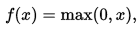, it returns 0 for all values below 0 and value itself for anything > 0. It’s much simpler in computation. Read more on “ReLU vs Sigmoind“.

Max pooling – pools the biggest value from the sample.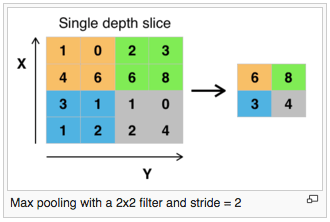Local response normalization –  permits the detection of high-frequency features with a big neuron response, while damping responses that are uniformly large in a local sample. Original description could be found in chapter “3.3 Local Response Normalization” here: http://papers.nips.cc/paper/4824-imagenet-classification-with-deep-convolutional-neural-networks.pdf). Additional reading: “Importance of local response normalization in CNN” | “What Is Local Response Normalization In Convolutional Neural Networks

These layers have recently fallen out of favor because in practice their contribution has been shown to be minimal” (Stanford CS class: CS231n Convolutional Neural Networks for Visual Recognition)

Learning rate schedule – applies exponential decay to the learning rate. Computed as: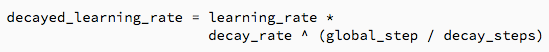(https://www.tensorflow.org/versions/r0.11/api_docs/python/train.html#exponential_decay)

Threading and Queues – mechanism for asynchronous computation. Threading and Queues in TensorFlow.

Softmax classifier – provides “probabilities” for each class.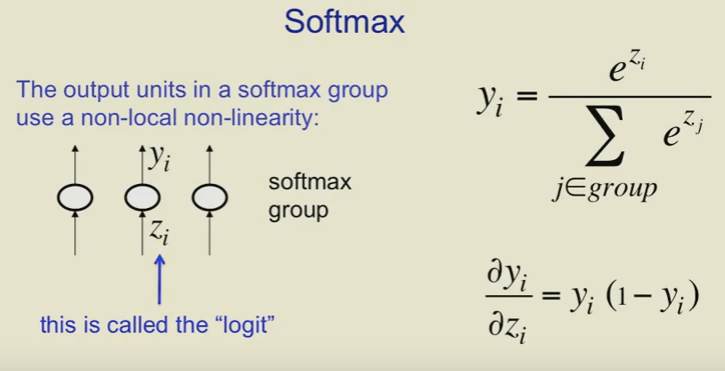Read more on softmax: http://cs231n.github.io/linear-classify/#softmax | Full playlist: https://www.youtube.com/watch?v=mlaLLQofmR8&list=PLoRl3Ht4JOcdU872GhiYWf6jwrk_SNhz9&index=18 | SVM vs SoftMax (CS231n)

### Files in this tutorial

• cifar10_input.py – processes the CIFAR-10 binary file format
• cifar10.py – builds the TensorFlow CIFAR-10 model
• cifar10_train.py – trains a TensorFlow CIFAR-10 model
• cifar10_eval.py – evaluates the performance of model

### cifar10_input.py – processing the binary CIFAR-10

Methods:

read_cifar10(filename_queue) – reads and parse examples from data files (from ‘filename_queue’), returns object representing the single example (fields: height, width, depth, key, label, uint8image)

_generate_image_and_label_batch(image, label, min_queue_examples, batch_size, shuffle) – constructs shuffled (if shuffle == true) queue of images with labels, returns images (4D tensor: batch_size, height, width, depth), and labels (1d tensor: batch_size).

distorted_inputs(data_dir, batch_size) – construct distorted input, returns distorted images and labels. Distortions applied to the image: crop; flip the image horizontally; brightness change, contrast change.

inputs(eval_data, data_dir, batch_size) – construct input for CIFAR evaluation.

libraries used:
numpy – scientific computing with Python
six.moves – support codebases towork on Python 2 and  Python 3
from tensorflow.models.image.cifar10 import cifar10” shall be replaced with “import cifar10” if you want to modify the code.

### cifar10.py – builds the model

from tensorflow.models.image.cifar10 import cifar10_input” shall be replaced with “import cifar10_input“: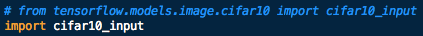Default flags:• data_dir (path to the CIFAR-10 data directory): ‘/tmp/cifar10_data’
• batch_size (number of images to process in a batch): 128
• use_fp16 (train the model using fp16): False

defined here: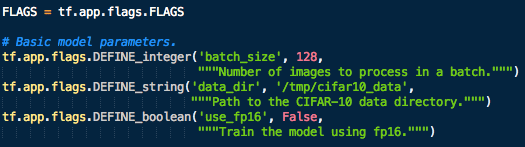Global constants describing the CIFAR-10 dataset:

```IMAGE_SIZE: 24 | <type 'int'>
NUM_CLASSES: 10 | <type 'int'>
NUM_EXAMPLES_PER_EPOCH_FOR_TRAIN: 50000 | <type 'int'>
NUM_EXAMPLES_PER_EPOCH_FOR_EVAL: 10000 | <type 'int'>```

defined here: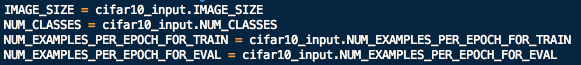and in cifar10_input.py:Constants describing the training process:

```MOVING_AVERAGE_DECAY: 0.9999 | <type 'float'>
NUM_EPOCHS_PER_DECAY: 350.0 | <type 'float'>
LEARNING_RATE_DECAY_FACTOR: 0.1 | <type 'float'>
INITIAL_LEARNING_RATE: 0.1 | <type 'float'>```

defined here: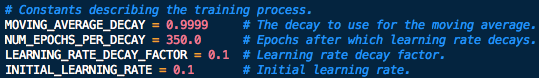```TOWER_NAME = 'tower'
DATA_URL = 'http://www.cs.toronto.edu/~kriz/cifar-10-binary.tar.gz'```

Methods:

_activation_summary(x) – helper creates summaries for activations of tensor ‘x’, provides  a histogram and measures the sparsity of activations.

_variable_on_cpu(name, shape, initializer) – helper creates and returns a Variable stored on CPU memory with following params: name (name of the variable), shape (list of integers), and initializer (initializer for Variable). dtape (DataType) is set to float32 if use_fp16==False.

_variable_with_weight_decay(name, shape, stddev, wd) – helper creates and returns an initialized Variable with weight decay with following params: name (name of the variable), shape (list of integers), stddev (standard deviation of a truncated Gaussian), and wd (L2Loss weight decay will be multiplied by this float, if == None, no weight decay). dtape (DataType) is set to float32 if use_fp16==False. Reference material: Truncated normal distribution (wiki).

distorted_inputs() – construct distorted input for CIFAR training, returns distorted images and labels. See ‘cifar10_input.py/distorted_inputs‘ for details. Returns images (4D tensor: batch_size, height, width, depth), and labels (1d tensor: batch_size).inputs(eval_data) – constructs input for CIFAR evaluation with ‘eval_data’.inference(images) – builds the TensorFlow CIFAR-10 model with ‘images‘ from distorted_inputs or inputs, returns softmax_linear.

This CIFAR-10 model consist from the following layers:

• `conv1 – 2D convolution and rectified linear activation (Example of 2D Convolution)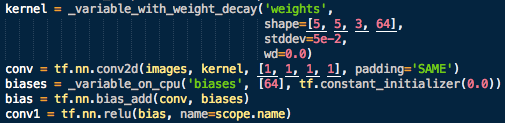`
• `pool1 – max pooling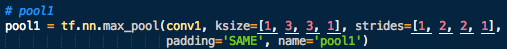`
• `norm1 – Local Response Normalization`
• `conv2 (same as conv1)`
• `norm2 (same as norm1)`
• `pool2 (same as pool1)`
• `local3 – fully connected layer with rectified linear activation`
• `local4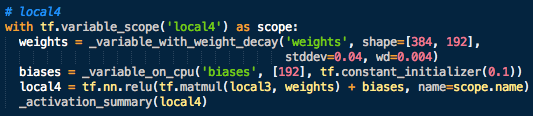`
• `softmax_linear – Softmax classifier (see explanation above).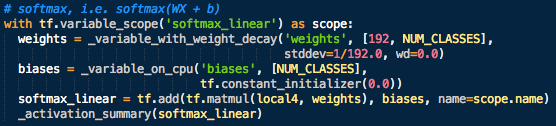`

loss(logits, labels) – Add L2Loss (summary of loss and loss/avg) to all the trainable variables, uses logits (logits from inference), and labels from ‘distorted_inputs‘ or ‘inputs‘. Read more on loss function in machine learning.

_add_loss_summaries(total_loss) – generates average for all losses and summaries for visualizing the performance of the network. Called from ‘train’:train(total_loss, global_step) – trains TensorFlow CIFAR-10 model: creates an optimizer and applies to all trainable variables, adds moving averages for all trainable variables, uses ‘total_loss’ (‘loss’) and ‘global_step’ (Integer, the number of training steps processed). Decays the learning rate (‘LEARNING_RATE_DECAY_FACTOR‘).Generates moving averages of all losses (‘total_loss‘). Computes and applies gradients (‘tf.train.GradientDescentOptimizer(lr)‘ and ‘tf.train.GradientDescentOptimizer(lr).apply_gradients(tf.train.GradientDescentOptimizer(lr).compute_gradients(total_loss), global_step=global_step)‘).  Creates histograms for trainable variables and gradients. Tracks the moving averages of  variables.

### cifar10_train.py – Training the TensorFlow CIFAR-10 model

Uses “six.moves” and “tensorflow” libraries.

from tensorflow.models.image.cifar10 import cifar10” shall be replaced with “import cifar10” if you want to make changes in the file.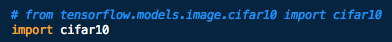Cifar-10 training – default flags:

• train_dir (directory where to write event logs and checkpoint): ‘/tmp/cifar10_train
• max_steps (number of batches to run): 1’000’000
• log_device_placement (whether to log device placement): False (‘log_device_placement‘ set to ‘True’ helps to find out which devices your operations and tensors are assigned to, used in Multi GPU Computations in TensorFlow).

main(argv=None) – checks if ‘FLAGS.train_dir’ directory exists, if not, creates the directory and downloads the dataset, otherwise runs the train().

train() – the main function of Cifar-10 training, trains for a number of steps specified in ‘FLAGS.max_steps‘ (Default value: 1’000’000).

• Gets dataset (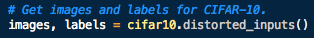).
• Builds a Graph that computes the logits predictions from the inference model ‘cifar10.inference(images)‘.
• Calculates ‘loss‘ (cifar10.loss(logits, labels)). Defines train_op (train_op = cifar10.train(loss, global_step)).
• Creates saver (tf.train.Saver) which saves and restores (if required) all variables:.
• Builds a summary operation based on TensorFlow collection of Summaries (‘summary_op’).
• Builds an initialization operation init:.
• Creates and runs a ‘tf.Session‘ (sess.run), with ‘init‘ and ‘config=tf.ConfigProto(log_device_placement=FLAGS.log_device_placement)‘.
• Starts threads for all queue runners collected in the graph and returns the list of all threads.
• Writes the summary protocol buffers with ‘tf.train.SummaryWriter‘ to event files.
• Runs the ‘tf.Session‘ with ‘train_op‘ and ‘loss‘ functions:in the loop until  ‘FLAGS.max_steps’ (1’000’000 steps) will be reached.
• Periodically reports (every 10th step) and saves (every 1000th step) the model checkpoints.

### cifar10_eval.py – evaluating the performance of TensorFlow CIFAR-10 model

Cifar-10 evaluation – default flags:

• eval_dir (directory where to write event logs and checkpoint): ‘/tmp/cifar10_eval
• eval_data (either ‘test’ or ‘train_eval’): ‘test’
• checkpoint_dir (where to read model checkpoints): ‘/tmp/cifar10_train
•  eval_interval_secs (how often to run the evaluation) – every 5 minutes (60*5)
•  num_examples (number of examples to evaluate) – 10’000
• run_once (if True will run the evaluation only once) – False

main(argv=None) – checks if ‘FLAGS.eval_dir’ directory exists, if required creates the directory and runs the evaluate().

eval_once(saver, summary_writer, top_k_op, summary_op) – runs one evaluation:

• Checks if any checkpoints are recorded in FLAGS.checkpoint_dir (‘/tmp/cifar10_train’). If none found, returns ‘No checkpoint file found‘. If you are getting this error make sure that you run the ‘cifar10_train.py’ for at least 1’000 steps.
• Starts the queue runners (Read more: TensorFlow Coordinator and QueueRunner) which counts the number of correct predictions and computes the precision.

evaluate() – evaluate TensorFlow CIFAR-10 model for a number of steps.

• Gets labels and images
• Builds a Graph that computes the logits predictions from the inference model
• Calculates predictions
• Restores the moving average version of the learned variables for evaluation
• Builds the summary operation based on the TF collection of Summaries